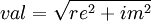# NMRPipe:MC magnitude calculation

(Redirected from NMRPipe:MC)

Function for magnitude calculation is MC. For each given data point it will calculate new value as:$val = \sqrt{re^2 + im^2}$

Example:

nmrPipe -fn  MC